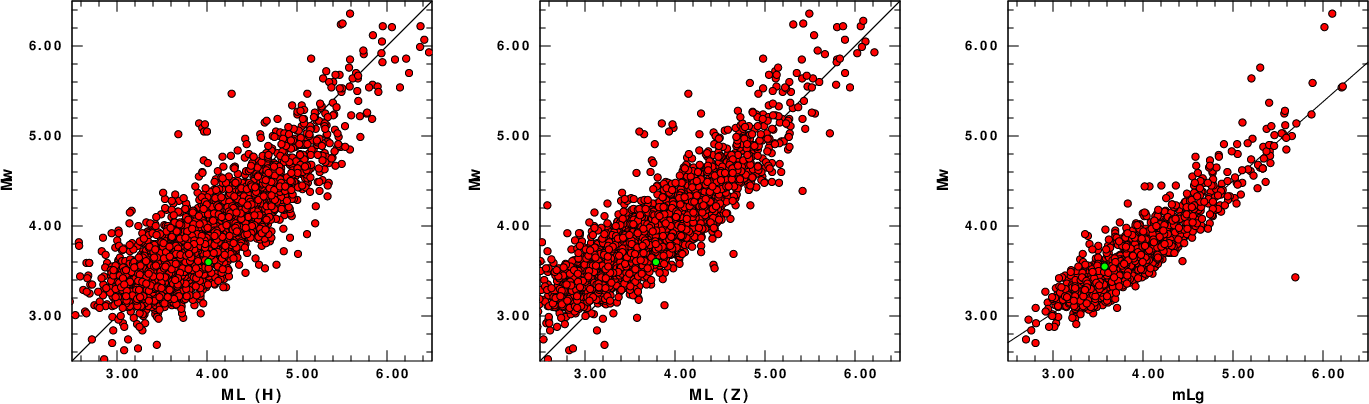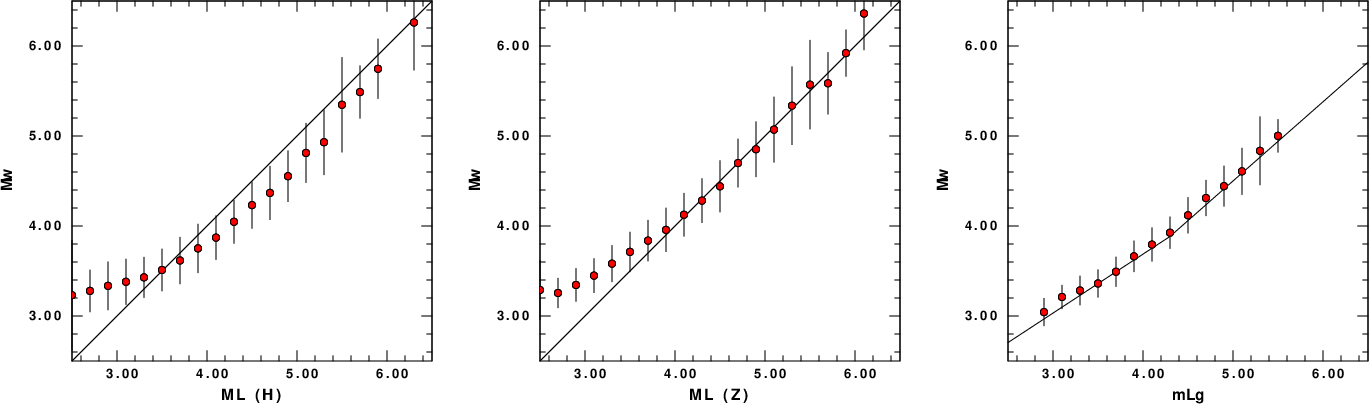## Magnitude

Although moment magnitude, Mw, is preferred to document an earthquake, other magnitudes are useful because they can be estimated for events too small for determining the usual broadband moment magnitude and, more importantly, automatically from a few observations before the moment tensor processing can begin. The ML and mLg magnitudes have been used in the United States for earthquakes west and east of the Rocky mountains, respectively. Starting in 2015, and for a few earlier selected events, the SLU moment tensor documentation includes an estimate of ML for all events, and mLg for eastern events.

The SLU determination of ML uses the NEIC methodology of preparing waveforms, and first applies the IASPEI (appropriate for Southern California) definition

ML = log A(nm) + 1.11 log R + 0.00189 R -2.09

where R is the hypocentral distance in km and A is the one-half maximum peak-to-peak horizontal amplitude of the S-wave signal of a simulated Wood-Anderson instrument with unit gain.

The SLU ML examines the trend of the individual ML estimates with distance and modifies the 0.00189 term to remove the distance trend. The other terms of the IASPEI formula are not changed. The rationale for this choice is to ensure that the same observed amplitude at short distance in Southern California and elsewhere would give the same magnitude. The modification of the term linear in R is thus assumed to document regional variations in the attenuation of high frequency motion.

For future reference the determinations for each event have been preserved the the file mwmlgml.txt which has a simple format:

```20170109203959 MW= 3.22 MLG= 3.421552 MLH= 3.312174 MLZ= 2.752702 LAT= 36.699 LON= -97.669 H= 2.0 NEWSLOPEH= -0.0002706 NEWSLOPEZ= -0.00063072 STK= 155 DIP= 75 RAKE= -10 MODEL= WUS EVID NONE VP0= 3.41 VS0= 2.01 R0= 2.21 VPD= 5.54 VSD= 3.30 RD= 2.61 REGIME= SS
20170118080432 MW= 3.58 MLG= -12345 MLH= 3.556398 MLZ= 3.406047 LAT= 38.407 LON= -118.929 H= 10.0 NEWSLOPEH= 0.00189508 NEWSLOPEZ= 0.00194679 STK= 210 DIP= 70 RAKE= -20 MODEL= WUS EVID NONE VP0= 3.41 VS0= 2.01 R0= 2.21 VPD= 6.27 VSD= 3.74 RD= 2.78 REGIME= SS
20170131093837 MW= 5.15 MLG= -12345 MLH= 5.116269 MLZ= 4.702123 LAT= 63.073 LON= -150.949 H= 130.0 NEWSLOPEH= 0.00155072 NEWSLOPEZ= 0.00062047 STK= 45 DIP= 80 RAKE= 70 MODEL= WUS EVID NONE VP0= 3.41 VS0= 2.01 R0= 2.21 VPD= 7.90 VSD= 4.62 RD= 3.28 REGIME= XX
20170213071712 MW= 5.21 MLG= -12345 MLH= 5.326130 MLZ= 4.911536 LAT= 62.515 LON= -142.749 H= 14.0 NEWSLOPEH= 0.00161754 NEWSLOPEZ= 0.000653 STK= 50 DIP= 90 RAKE= -5 MODEL= WUS EVID NONE VP0= 3.41 VS0= 2.01 R0= 2.21 VPD= 6.27 VSD= 3.74 RD= 2.78 REGIME= SS
20170216153307 MW= 3.30 MLG= -12345 MLH= 3.952183 MLZ= 3.190272 LAT= 36.119 LON= -96.861 H= 5.0 NEWSLOPEH= 0.00086075 NEWSLOPEZ= -0.00060075 STK= 300 DIP= 85 RAKE= 30 MODEL= WUS EVID NONE VP0= 3.41 VS0= 2.01 R0= 2.21 VPD= 5.54 VSD= 3.30 RD= 2.61 REGIME= SS
20170218144039 MW= 3.89 MLG= -12345 MLH= 4.170510 MLZ= 3.464857 LAT= 50.871 LON= -124.300 H= 16.0 NEWSLOPEH= 0.00206213 NEWSLOPEZ= 0.00087719 STK= 190 DIP= 75 RAKE= -15 MODEL= WUS EVID NONE VP0= 3.41 VS0= 2.01 R0= 2.21 VPD= 6.27 VSD= 3.74 RD= 2.78 REGIME= SS
20170219221729 MW= 4.06 MLG= -12345 MLH= 4.317327 MLZ= 3.867061 LAT= 59.787 LON= -153.152 H= 120.0 NEWSLOPEH= 0.0027892 NEWSLOPEZ= 0.00190265 STK= 45 DIP= 70 RAKE= 20 MODEL= WUS EVID NONE VP0= 3.41 VS0= 2.01 R0= 2.21 VPD= 7.90 VSD= 4.62 RD= 3.28 REGIME= SS
20170223045903 MW= 3.98 MLG= -12345 MLH= 4.041532 MLZ= 3.942498 LAT= 47.486 LON= -123.054 H= 17.0 NEWSLOPEH= 0.00194281 NEWSLOPEZ= 0.00228366 STK= 60 DIP= 50 RAKE= 35 MODEL= WUS EVID NONE VP0= 3.41 VS0= 2.01 R0= 2.21 VPD= 6.27 VSD= 3.74 RD= 2.78 REGIME= TS
```
The columns are the event time, followed by values determined. If mLg is not determined (usually for events in the West), the column has a placeholder value of -12345. The outut for the first event has the following meaning:
```20170109203959        Origin time in YearMonthDayHourMinuteSecond
MW= 3.22              Mw determined for this event using the WUS model
MLG= 3.421552         mLg computed using IASPEI formula
A value of -12345 inicates that this magnitude was not determined
MLH= 3.312174         ML from horizontal computed using methodology described here
A value of -12345 inicates that this magnitude was not determined
MLZ= 2.752702         ML from vertical component using methodology describe here. This is for reasearch
A value of -12345 inicates that this magnitude was not determined
LAT= 36.699           Event latitude
LON= -97.669          Event longitude
H= 2.0                Event depth
NEWSLOPEH= -0.0002706 New slope for ML formula based on horizontal data.
ML = log A(nm) + 1.11 log R - 0.00027 R -2.09
NEWSLOPEZ= -0.00063072 New slope for ML formula based on vertical data (for research)
ML = log A(nm) + 1.11 log R - 0.00063 R -2.09
STK= 155              Mechanism strike
DIP= 75               Mechanism dip
RAKE= -10             Mechanism rake
MODEL= WUS            WUS velocity model
See description at  http://www.eas.slu.edu/eqc/eqc_mt/MECH.NA/MECHFIG/mech.html
EVID NONE             Event ID
VP0= 3.41             P-velocity at surface (km/s) for this model
VS0= 2.01             S-velocity at surface (km/s)
R0= 2.21              Density at surface (gm/cm^3)
VPD= 5.54             P-velocity at source depth (km/s)
VSD= 3.30             S-velocity at source depth (km/s)
RD= 2.61              Density at source depth (gm/cm^3)
REGIME= SS            World stress map classification
The symbols describing the regime are from Zoback (1992):
NF - normal fault,
SS - strike-slip fault,
TF - thrust fault,
NS - normal-strike slip oblique fault,
TS - thrust-strike-clip oblique fault,
XX - no clear determination of direction of maximum compressive stress.
```
ML is estimated using the Horizontal (H) and Vertical (Z) components. For each the newslope in the magnitude equation is givn. Thus the entry for the earthquake of 2017/01/09 20:39:59 indicates that the ML(H) formula there should be

ML = log A(nm) + 1.11 log R - 0.00063 R -2.09

The reason for all of this information is to permit future studies on the regional veriation of the slope term, and a detailed study of the Mw(ML) relation in terms of source mechanism and other parameters that may explain the scatter in the Mw(ML) relation.

## Relation between moment magnitude and local magnitude

the following figures presents Mw vs ML for all earthquakes and Mw vs mLg for which the values have been computed. The value of the last earthquake in the list is given by a green colored symbol.

 Raw Mw vs local magnitude determinationsCLICK ON THE IMAGE FOR INTERACTIVE VIEWING One sigma bounds on Mw's for local magnitude binsPlot showing mean magnitude and 1 standard deviation bars

## Reformatting

To reformat the mwmlgml.txt into a CSV tab-separated format for use with a spreadsheet, do the following:
```cat mwmlgml.txt | awk '{printf "%s\t%5.2f\t%14.2f\t%14.2f\t%14.2f\t%6.3f\t%8.3f\t%3.0f\t%8.5f\t%8.5f\t%d\t%d\t%d\t%s\t%s\t%f\t%f\t%f\t%f\t%f\t%f\t%s\n",\$1,\$3,\$5,\$7,\$9,\$11,\$13,\$15,\$17,\$19,\$21,\$23,\$25,\$27,\$29,\$31,\$33,\$35,\$37,\$39,\$41,\$43}' > mwmlgml.csv
```

to obtain

```20170109203959	 3.22	          3.42	          3.31	          2.75	36.699	 -97.669	  2	-0.00027	-0.00063	155	75	-10	WUS	NONE	3.410000	2.010000	2.210000	5.540000	3.300000	2.610000	SS
20170118080432	 3.58	     -12345.00	          3.56	          3.41	38.407	-118.929	 10	 0.00190	 0.00195	210	70	-20	WUS	NONE	3.410000	2.010000	2.210000	6.270000	3.740000	2.780000	SS
20170131093837	 5.15	     -12345.00	          5.12	          4.70	63.073	-150.949	130	 0.00155	 0.00062	45	80	70	WUS	NONE	3.410000	2.010000	2.210000	7.900000	4.620000	3.280000	XX
20170213071712	 5.21	     -12345.00	          5.33	          4.91	62.515	-142.749	 14	 0.00162	 0.00065	50	90	-5	WUS	NONE	3.410000	2.010000	2.210000	6.270000	3.740000	2.780000	SS
20170216153307	 3.30	     -12345.00	          3.95	          3.19	36.119	 -96.861	  5	 0.00086	-0.00060	300	85	30	WUS	NONE	3.410000	2.010000	2.210000	5.540000	3.300000	2.610000	SS
20170218144039	 3.89	     -12345.00	          4.17	          3.46	50.871	-124.300	 16	 0.00206	 0.00088	190	75	-15	WUS	NONE	3.410000	2.010000	2.210000	6.270000	3.740000	2.780000	SS
20170219221729	 4.06	     -12345.00	          4.32	          3.87	59.787	-153.152	120	 0.00279	 0.00190	45	70	20	WUS	NONE	3.410000	2.010000	2.210000	7.900000	4.620000	3.280000	SS
20170223045903	 3.98	     -12345.00	          4.04	          3.94	47.486	-123.054	 17	 0.00194	 0.00228	60	50	35	WUS	NONE	3.410000	2.010000	2.210000	6.270000	3.740000	2.780000	TS
```